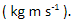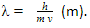# Wave-Particle Duality

## Introduction

Light waves can behave like particles (photons) and waves. This phenomena is called the wave-particle nature of light or wave-particle duality. In this article, how a light wave behaves like a particle and a wave are explained.

A. Diffraction

When a beam of X-rays of a single wavelength is directed at a thin metal foil, a diffraction pattern is produced, as illustrated in Figure 1. This phenomenon is similar to the diffraction pattern produced when light passes through a diffraction grating. The foil contains many tiny crystals. The gaps between neigbouring planes of the atoms in the crystals act as slits, creating a diffraction pattern.

Electrons are considered to be particles. When a beam of electrons is directed at a graphite film, a similar diffraction pattern is produced, as shown in Figure 1.Figure 1: Diffraction Pattern of Waves and Particles

B. Photoelectric Effect

According to Albert Einstein’s theory of quantised energy, light energy consists of a stream of energy packets called photos. A photon is defined as a quantum of energy when the energy is in the form of electromagnetic radiation. Photoelectric emission is defined as the release of electrons from the surface of a metal when electromagnetic radiation is incident on its surface. The electrons that are released in the photoelectric emission are called photoelectrons. This shows the particle nature of light.

C. Momentum of a Particle

The momentum of a particle can be calculated by multiplying its mass in kilograms (kg) by its velocity in metres per second. Momentum is measured in kilogram metres per secondIn equation form,D. De Broglie’s Wavelength

In 1924, the French scientist Louis de Broglie suggested that all moving particles have a wave-like nature. Using ideas based upon the quantum theory and Einstein’s theory of relativity, he suggested that the momentum (p) of a particle and its associated wavelength (λ) are related by the following equation:E. Summary

• Light waves can behave like particles (photons) and waves. This phenomena is called the wave-particle nature of light or wave-particle duality.
• A diffraction pattern is produced when a beam of X-rays of a single wavelength is directed at a thin metal foil.
• Electrons are considered to be particles. When a beam of electrons is directed at a graphite film, a diffraction pattern is produced.
• Photoelectric emission shows that light can behave like a particle.
• The momentum of a particle can be calculated by multiplying its mass in kilograms (kg) by its velocity in metres per second• Scientist Louis de Broglie suggested that all moving particles have a wave-like nature. De Broglie’s wavelength of a moving particle is written as